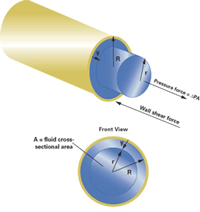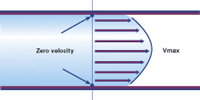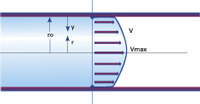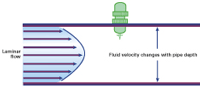# Velocity-Profile Deviations Influence Flowmeter Performance

## Velocity-Profile DeviationsInfluence Flowmeter Performance

#### Selecting the right flowmeter for the liquid

By Corte Swearingen
Newtonian Velocity Profiles    Non-Newtonian Fluids
Flowmeter Performance and Flow Profile Distortions
Assessing Pipe Flow Conditions    Flow Conditioners
Conclusion and Recommendations    References

Close your eyes and imagine a world in which all fluids flowing down pipes are perfectly homogeneous, having no disturbances or eddies in the flow stream. A world in which pipes always contain fully developed turbulent flow and pipe bends and obstructions are non-existent.

Sounds silly right? But these are the hypothetical conditions upon which flowmeter accuracies are based. If deviations from these conditions exist, accuracy and/or repeatability will degrade. This article will discuss the first of four major influence quantities that affect flowmeter accuracy and performance: velocity-profile deviations, non-homogenous flow, pulsating flow, and cavitation. With this background, we will then look at how these influence quantities factor into flowmeter performance and what can be done to minimize their effect.

Imagine a horizontal pipe a few feet in front of you at about eye level. If a vertical cut were made through the center of the pipe to remove the front half of the pipe, it would be easy to see how "nicely behaved" fluid flow develops.Figure 1: Zero Liquid ViscosityIn the hypothetical situation shown here, the liquid viscosity is zero, and the velocity profile is a straight line. The vertical sheet of fluid moves forward at velocity V.

The cross-sectional view shown in Figure 1 illustrates a hypothetical situation in which the fluid has zero viscosity. In this example, the velocity gradient is constant and equal across the entire cross-section. That is, the fluid velocity at the pipe walls is equal to the fluid velocity at the pipe center and at all points in-between. However, every liquid has some measure of viscosity; therefore, the velocity profile becomes distorted from this "ideal case scenario."

Newtonian Velocity Profiles

Examples of Newtonian fluids include water, milk, sugar solutions and mineral oils. Distortions can occur for homogeneous Newtonian fluids for a number of reasons. The viscosity of a Newtonian fluid is dependent only on temperature, but not on shear rate and time. The flow of a Newtonian fluid will exhibit a "sticking" effect on the walls of the pipe. In fact, the boundary conditions placed on the stationary pipe wall demand that the fluid in direct contact with the pipe walls have zero velocity (or at least that is what is assumed in the calculations within this article). It is this boundary condition that distorts a Newtonian fluid's velocity profile.

If the velocity vector at the pipe wall is zero, the maximum velocity occurring in the pipe can be worked out mathematically.Figure 2: Sample Newtonian Fluid Velocity ProfileFor steady flow in a pipe of radius "R" containing a smaller cylindrical element of liquid radius "r," a shear force develops equal and opposite to the cylindrical water element pressure.

Consider a pipe of radius "R" that contains a smaller cylindrical element of liquid of radius "r." See Figure 2.

Assuming the cylindrical element of fluid is moving uniformly through the pipe, at constant velocity, the shearing force on the pipe wall is equal to the pressure force of the liquid moving through. The shear force, which is directly proportional to the velocity gradient, is given by:

τ = μ(dv/dy)

where:
τ is the shear force or shear pressure.
dv/dy is the velocity gradient (where y is the distance from the pipe wall).
μ is the constant of proportionality, also referred to as the dynamic viscosity.

Because the fluid pressure can be simply given as the pressure differential through the pipe times the cross-sectional fluid area, and because the fluid pressure force is equal to the oppositely directed shear force on the pipe wall, the equation can be written:

Shear Wall Force = τ(2πr)L = 2πrLμ(dv/dy) = ΔPA = ΔPπr2 = Fluid Pressure

where:
r is the radius of the cylindrical fluid element.
L is the length of the cylindrical fluid element.
ΔP is the pressure differential through the pipe.
A is the cross-sectional area of the fluid element

Solving for τ, we get

τ = ΔPr/2L

It is more convenient in this example to give the shear force as a function of the pipe center instead of the pipe wall. This is easily accomplished easily by rewriting the shear force as:

τ = -μ(dv/dr) = μ(dv/dy)

Equating these two expressions for the shear stress τ gives us the following relationship:

ΔPr/2L = -μ(dv/dr)

Solving for the velocity gradient yields:

dv/dr = -ΔPr/2μL

By integrating this expression across the pipe to radius R, the velocity (V) as a function of the radius r can be determined.

V = ΔP/4μL(R2 - r2)

Now it can be seen mathematically that the maximum velocity in the pipe occurs at the center of the pipe, or when r = 0. Actually, these two factsthat the velocity at the pipe wall is zero and the maximum velocity occurs at the pipe centerare the boundary conditions used to determine the constant of proportionality for the above integration.

This equation is in the form of a parabola. Graphing it gives the velocity profile shown in Figure 3.Figure 3: Laminar Flow Profile for Newtonian FluidThe velocity is zero at the pipe wall and increases parabolically with flow, reaching its maximum at the pipe's center.

This profile develops at certain velocities for Newtonian fluids and is called laminar flow. Laminar flow is characterized by fluid movement in layers or streamlines with very little mixing occurring between those layers. The fluid layers are restrained from mixing by the viscous forces within the liquid.

Laminar flow is very well behaved and predictable. However, as the velocity of the fluid increases within the pipe, inertial forces start to overcome the viscous forces, and small eddies and fluid oscillations will form, causing the fluid layers to start mixing.

For a while, the fluid might shift to being somewhat laminar, but the eddies keep working to mix the fluid layers. This partial mixing of the streamlined layers is known as transitional flow.

Transitional flow is not really laminar flow but it is not fully turbulent flow either. In fact, the fluid may exhibit laminar flow at some pipe radii and turbulent flow at other radii. If a dye stream were to be injected into the pipe, it would waver and mix slightly as it moves down the pipe. The velocity profile for transitional flow is difficult to predict because it changes with time, being laminar-like in some instances and turbulent-like in others.

As the velocity of the fluid within the pipe increases further, fully turbulent flow develops. Turbulent flow is characterized by the laminar flow streams completely breaking up and mixing together as the flow moves down the pipe. Although the average flow still moves down the pipe, there will be small flow velocities in the radial r direction as well. As eddies form in the fluid stream, they will break down randomly into smaller eddies, swirls and vortices, leading to increased shear within the liquid.

Is there any way to predict when each of these regimeslaminar, transitional and turbulent flowwill dominate the fluid flow? The answer, of course, is yes. The Reynolds number helps determine what type of flow can be expected. Named after the fluid dynamics research of Osborne Reynolds, the Reynolds number is simply the ratio of momentum to viscous forces. It takes into account the fluid density, viscosity, and pipeline velocity. If the viscous forces are large (low Reynolds number), the viscous effects of the fluid will dampen out any tendency of streamline mixing. For large inertial forces, there will not be enough viscous damping for laminar flow to continue, and the flow will become transitional or turbulent. The equation for the Reynolds number is:

R = vDρ/μ

where:
v is the average velocity.
D is the pipe diameter.
ρ is the density.
μ is the dynamic viscosity.

Using SI units, it becomes apparent that the Reynolds number is dimensionless. The units of vDρ are (meters/sec) x (meters) x (kg/meters3), and the units of μ are (kg/meters x sec). The rule of thumb is that the flow will be laminar below an R of 2,000. Between an R of 2,000 and 4,000, the flow is transitional; above 4,000, the flow is turbulent. In actuality, there are no distinct breaks between laminar, transitional, and turbulent flows. For example, depending on the fluid, fully developed transitional flow might not occur until R equals roughly 7,000.

So, what is the velocity profile for turbulent flow? This is a difficult question as the profile is not a fixed geometry. It changes with the wall roughness and Reynolds number. The velocity equation for homogeneous Newtonian flow in the turbulent regime (see Figure 4) is:

Vr = Vav[1 1.44√f 2.15√f log10(1-r/R)]

where:
Vr is the velocity at radius r.
Vav is the average flow velocity.
R is the radius of the pipe.
f is the friction factor.

The friction factor is difficult to calculate because turbulent flow is unpredictable, but a lot of experimental data exist on this subject. The friction factor comes into play when the pipe wall is not smooth. For a rough pipe interior, the fluid closer to the walls is held back because of the additional shear. This, in turn, causes the velocity profile to become flatter and blunter in front.Figure 4: Turbulent Flow Profile for a Newtonian FluidThe velocity is zero at the pipe wall, but the face velocity is straighter and squared up.

One widely used method of determining the friction factor is the Moody diagram. Moody-diagram-related references are included in the bibliography.

The velocity equation shows that the maximum flow will occur at r = 0, just like it did for laminar flow. At r = 0, the equation becomes:

Vmax = Vav (1 1.43√f)

Figure 4 shows that the face velocity is more uniform and squared up. As the velocity of the fluid continues to increases, the face velocity will continue to straighten up until all particles are moving at the same velocity (except at the pipe walls, where the flow will remain at zero). This hypothetical situation, where the face velocity is completely flat, is known as infinite Reynolds number profile, or plug flow.

Non-Newtonian Fluids

How does the velocity profile change when the ratio of shear stress to shear strain is no longer constant? Before attempting to answer this question, it is helpful to outline the different types of non-Newtonian fluids.

Class I: time-independent non-Newtonian fluids. These fluids have a viscosity at a given shear stress that does not vary with time. Class 1 nonNewtonian fluids include pseudoplastic, dilatant and plastic fluids.

Pseudoplastic fluids, also known as shear-thinning fluids, decrease in viscosity as the shear rate increases. Examples include paints, shampoos and water suspensions of clay.

Dilatant fluids, also known as shear-thickening fluids, increase in viscosity as the shear rate increases. Examples include corn starch in water, titanium dioxide, and wet sand.

Plastic fluids, also called Bingham or plug-flow fluids, behave as a solid until a critical shear ratecalled the yield valueis reached. At the yield value, the fluid will start to flow. As the shear rate continues to increase, the fluid then might exhibit Newtonian, dilatent or pseudoplastic characteristics. Examples include tomato paste, toothpaste, hand cream, chocolate, mayonnaise, and grease.

Class II: time-dependent non-Newtonian fluids. These fluids have a viscosity at a given shear stress that will vary with time. They include thixotropic and rheopectic fluids.

Thixotropic fluids, also known as time-thinning fluids, experience a decrease in viscosity over time while the rate of shear is constant. Examples include yogurt and paint.

Rheopectic fluids, also called time-thickening fluids, exhibit an increase in viscosity over time as the shear remains constant. Rheopectic fluids are pretty rare. Examples include gypsum paste and printers ink.

What makes the flow of nonNewtonian fluids so interesting is the required boundary condition of zero flow at the pipe walls. This boundary condition imparts a varying shear rate into the fluid as the fluid velocity changes. And because a nonNewtonian fluid exhibits changing viscosity with changing shear rates, the fluid can react in a wide variety of ways. Like Newtonian fluids, non-Newtonian fluids have laminar, transitional, and turbulent flow patterns.

For time-independent nonNewtonian fluids, the fluid viscosity does not change with time, assuming a nonchanging shear rate. As the fluid moves down the pipe, the velocity distribution between the fluid layers varies and changes with the varying shear that results from the zero velocity boundary condition at the walls of the pipe.

Another important condition is the apparent viscosity of the liquid. Because the fluid viscosity changes with the shear rate, the apparent viscositythe viscosity at a particular rate of shearmust be used to calculate the flow profile instead of the dynamic or absolute viscosity discussed for Newtonian fluids. This makes the velocity profile impossible to predict completely and correctly.Figure 5: Time-Independent NonNewtonian Laminar Flow ProfilesShown are profiles of pseudoplastic, dilatant and plastic liquids over the profile of a Newtonian liquid.

However, the velocity profile still has some inherent symmetry. For the laminar flow region, the velocity profile is calculated as a deviation or departure from the laminar Newtonian profile. Figure 5 lays the typical velocity profiles of pseudoplastic, dilatant, and plastic liquids over the profile of a Newtonian liquid, revealing the distortions that can occur for time-independent nonNewtonian laminar flows.

The face profile for a plastic fluid is very flat. The entire flat face is known as the plug diameter and can vary greatly, depending on the type of plastic fluid moving down the pipe, as well as on the upstream pressure. Remember, plastic fluids will behave as a solid until shear stress reaches the critical yield value. They then will start flowing.

NonNewtonian fluids can be characterized by a Reynolds number whereby they will move from laminar to transitional to turbulent flows. This Reynolds number, however, is calculated differently for pseudoplastic, dilatant, and plastic fluids. For fully developed turbulent flow, flow profiles are similar enough to Newtonian fluids that the Newtonian Reynolds number equation can be used if apparent viscosity is substituted for dynamic viscosity.

Thixotropic fluids can experience some interesting effects, depending on whether the shear increases, decreases, or stays constant. A thixotropic fluid that increases in shear rate up to a constant value, dwelling at that value for a time before dropping back down to zero shear, can experience a decrease in apparent viscosity during the dwell time. When the shear rate is brought back down to zero, a yield value can be reached that will mimic the effects of a plastic fluid. The flow profile will go from parabolic shaped to plug-flow shaped. Of course, this type of pipeline scenario is extremely rare.

Because rheopectic fluids increase in apparent viscosity with time at constant shear, they are sometimes known as negative thixotropic fluids. Rheopectic fluids will exhibit the same type hysteresis curve as thixotropic fluids, but generally return to the same initial viscosity once the rate of shear drops to zero. Like thixotropic fluids, rheopectic fluids moving through a pipe are normally in the laminar regime and will typically exhibit the normal-to-slightly distorted parabolic curves already discussed, assuming that the flow has had enough time to fully develop through a straight section of pipe.

An excellent and much deeper discussion of flow prfiles and how they develop in pipe flow can be found in the Flow Measurement and Engineering Handbook, written by Richard Miller ( #00545-08).

Flowmeter Performance and Flow Profile Distortions

In each case discussed previously, the flow is through a long, straight section of piping, and the velocity profile had time to develop and stabilize as it moved down the pipe. This stabilization and development of the velocity profile is known as fully developed flow. Although it is great for doing fluid dynamics calculations, in the real world, pipes undergo bends, turns, reductions, and enlargements as they twist their way through a typical plant. What happens to these velocity profiles under some of these conditions, and how do the velocity profiles affect flowmeter placement and flowmeter performance?

In general, because the flow profile is smooth and flat over most of the pipe diameter, turbulent flow is preferred for a flowmeter over laminar and transitional flows. It's much easier to calibrate and check the repeatability of a flowmeter using a fully developed turbulent flow profile than it is using the parabolic flow profile of laminar flow or the unstable flow profile of transitional flow.

This is not mean laminar flow is bad for flowmeters. In fact, stable laminar flow is fairly easy to measure accurately, providing the appropriate technology is used.

The first step in choosing a particular flow technology is to find out its acceptable Reynolds number range and then to calculate the Reynolds extremes of the application. A minimum Reynolds number is calculated by using the minimum expected fluid flow and density and the maximum expected viscosity. A maximum Reynolds number is calculated using the maximum expected fluid flow and density and the minimum expected viscosity.

Another way to assess the conditions of a flow application is through use of Doppler or transit-time flow technologies.

Paddle-Wheel Flowmeters: These use a small rotor usually having four blades embedded with magnets. The paddle is inserted into the pipe at a fixed depth; the rate at which it turns is proportional to the flowrate.

One of the advantages of the paddle-wheel meter is it will work with a large range of pipe sizes. Typically, the paddle-wheel sensor can be installed in plastic or metal pipes from 1/2 inch (in.) up to 36 in. or larger. The paddlewheel itself is only an inch or so in diameter, so if it is installed in a large pipe, it would have problems measuring laminar flow (see Figure 6).Figure 6: Paddle-Wheel Flowmeter Installation ExampleAlthough they can be used in stable laminar flow applications to read maximum or average flow, paddle-wheel flowmeters are more accurate when used with fully developed turbulent flow.

Adjustable insertion depth sensors are available to allow exact positioning of the paddle-wheel within the pipe, but they have limited value with laminar flow and assume that the ratio between the measured velocity and the average fluid velocity is stable, which is not always the case. If an insertion sensor must be used in a laminar flow application, plant operators should try to find the point where the fluid velocity is related to the average velocity on a one-to-one basis. This point will be roughly one-eighth of the pipe diameter from the inside pipe wall, and should be fairly independent of the Reynolds number.

For smaller pipe sizes of a few inches or so, this is a lesser problem, and center-line insertion might be the best option. For a 20-inch pipe however, the flow profile gradient can be too sharp to allow accurate or consistent measurement by correlating a specific velocity within the pipe to an average pipe velocity. Similarly, for transitional flow, because the flow profile is unstable, the paddle-wheel design does not offer accurate or repeatable results. Because turbulent flow profile is blunted, flat and stableassuming it has had time to fully stabilize while flowing down the pipeit is the best profile when using a paddle-wheel sensor. In fact, manufacturers will calibrate their paddle sensors to fully developed turbulent flow within their flow calibration loop; therefore, paddle-wheel manufacturers have very specific upstream and downstream straight piping requirements.

Venturi-Cone Differential Pressure Flowmeters: These flowmeters offer quite a design contrast to paddle-wheel flowmeters. Developed in the early 1980's, the Venturi-cone, or V-cone for short, uses a cone positioned in the pipe's center. The cone geometry and placement force the fluid to move around the cone, generating a pressure drop across the length of the cone. See Figure 7. This pressure drop is proportional to the flow velocity within the pipe.Figure 7: Venturi-Cone Flowmeter Installation ExampleIn this installation, the suspended cone reshapes the approaching flow profile upstream.

The cone is always suspended at the pipe center, so for laminar flow, the maximum fluid flow is driven into the "point" of the cone. This effectively forces the high-velocity portion of the laminar flow stream to mix with the lower velocity layers, causing the upstream flow profile to mix and flatten into a more well-developed turbulent pattern.

The V-cone's ability to flatten irregular flow profiles is quite strong and even allows the V-cone meter to be installed fairly close to pipe elbows and bends, something usually avoided with other flow technologies. The meter acts as its own flow conditioner, shaping irregular or laminar flow profiles into more stable turbulent-like flow profiles. This allows the technology to be used over a wide range of Reynolds number.

Turbine Flowmeters: These flowmeters use a rotating turbine placed directly in the fluid path. Although designs vary, a pulsing signal usually is generated as the turbine blades pass a magnetic pickup coil. The frequency of the pulses is proportional to the fluid velocity in the pipe. For laminar and transitional flow patterns (i.e., having low Reynolds numbers), the number of pulses per unit volume, the K-factor, can vary, causing a loss of accuracy and repeatability. For this reason, turbine meters are best used in turbulent flow conditions. Many turbine manufacturers that build flow straighteners directly into the body of the flowmeter to minimize any upstream swirls generated by pipe bends, which also can degrade accuracy and repeatability. Insertion turbine meters, like the paddle-wheel sensors, will measure the velocity at only a particular point within the pipe. Therefore, they are better suited to fully developed turbulent flow.

Magnetic Flowmeters: These flowmeters measure the average flow over the pipe diameter and show very little performance change in going from laminar to transitional to fully turbulent flow. Insertion mag meters will measure the flow within a localized point around the sensor.

In both designs, however, swirling eddies or vortices that occur in pipe bends and turns can pose problems. Also, because velocity profile distortions can occur in several fluid planes simultaneously, velocity averaging for full-bore magnetic flowmeters has its limitations. For this reason, manufacturers usually recommend five pipe diameters of straight run pipe upstream and two pipe diameters downstream to stabilize any variations in the flow profile.

Gear Flowmeters: These flowmeters work by forcing specific volumes of fluid between two coupled gears. The fluid normally is trapped between the gear and the inner flowmeter housing. After the number of fluid pockets passing through in a particular time period is determined, the flow can be calculated. See Figu